# Threads What do we have so far n

• Slides: 35
Download presentation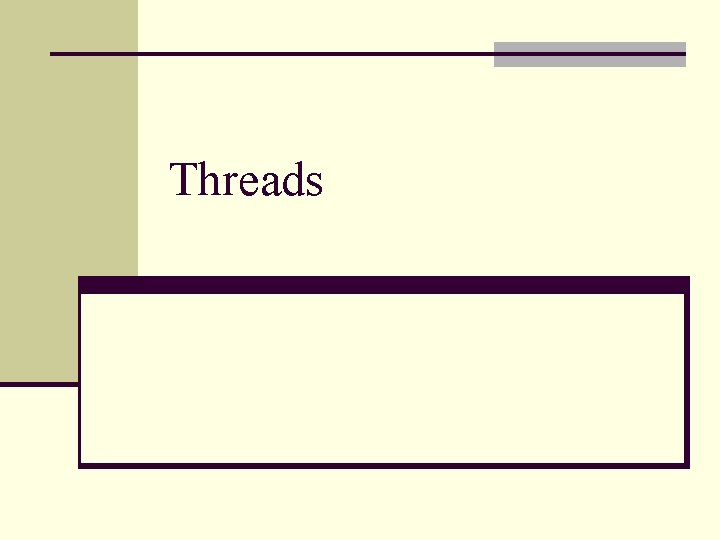ThreadsWhat do we have so far n The basic unit of CPU utilization is a process. n To run a program (a sequence of code), create a process. n Processes are well protected from one another. n Switching between processes is fairly expensive. n Communications between processes are done through inter-process communication mechanisms. n Running process requires the memory management system. n Process I/O is done by the I/O subsystem.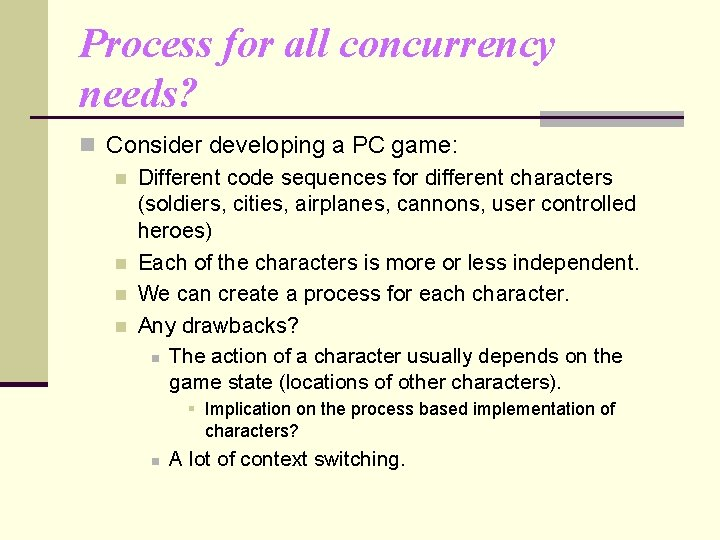Process for all concurrency needs? n Consider developing a PC game: n Different code sequences for different characters (soldiers, cities, airplanes, cannons, user controlled heroes) n Each of the characters is more or less independent. n We can create a process for each character. n Any drawbacks? n The action of a character usually depends on the game state (locations of other characters). § Implication on the process based implementation of characters? n A lot of context switching.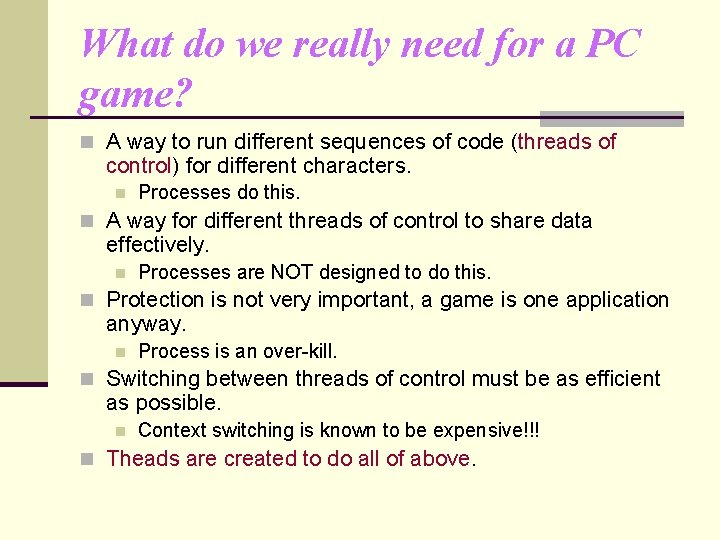What do we really need for a PC game? n A way to run different sequences of code (threads of control) for different characters. n Processes do this. n A way for different threads of control to share data effectively. n Processes are NOT designed to do this. n Protection is not very important, a game is one application anyway. n Process is an over-kill. n Switching between threads of control must be as efficient as possible. n Context switching is known to be expensive!!! n Theads are created to do all of above.Thread n Process context Process ID, process group ID, user ID, and group ID n Environment n Working directory. n Program instructions n Registers (including PC) n Stack n Heap n File descriptors n Signal actions n Shared libraries n Inter-process communication tools n What is absolutely needed to run a sequence of code (a thread of control)? nProcess/Thread context n What are absolutely needed to support a stream of instructions, given the process context? n n n Process ID, process group ID, user ID, and group ID Environment Working directory. Program instructions Registers (including PC) Stack Heap File descriptors Signal actions Shared libraries Inter-process communication tools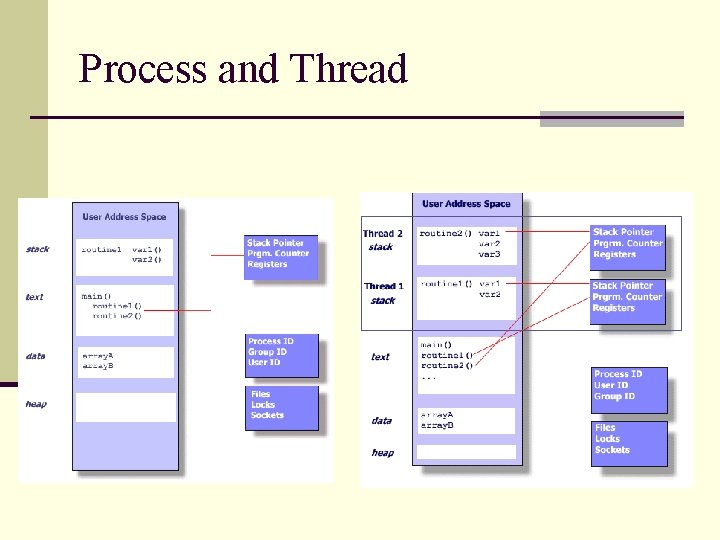Process and Thread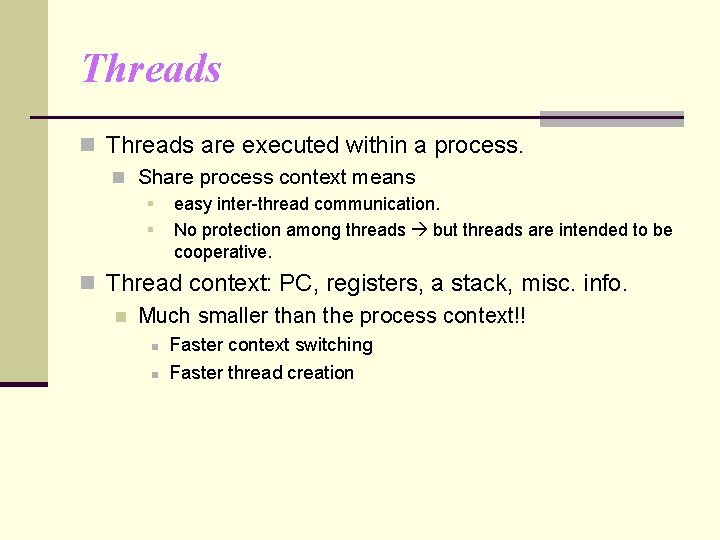Threads n Threads are executed within a process. n Share process context means § § easy inter-thread communication. No protection among threads but threads are intended to be cooperative. n Thread context: PC, registers, a stack, misc. info. n Much smaller than the process context!! n n Faster context switching Faster thread creationThreads n Threads are also called light-weight processes. n traditional processes are considered heavy-weight. n A process can be single-threaded or multithreaded. n Threads become so ubiquitous that almost all modern computing systems use thread as the basic unit of CPU utilization.More about threads n OS view: A thread is an independent stream of instructions that can be scheduled to run by the OS. n Software developer view: a thread can be considered as a “procedure” that runs independently from the main program. n n Sequential program: a single stream of instructions in a program. Multi-threaded program: a program with multiple streams n Multiple threads are needed to use multiple cores/CPUs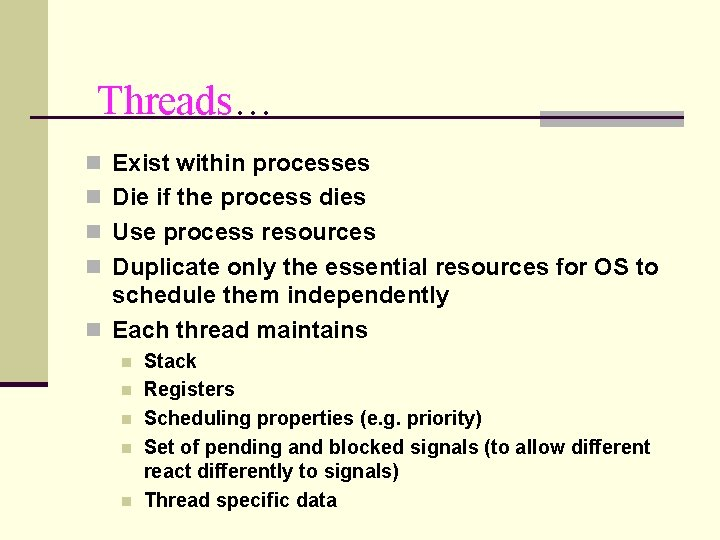Threads… n Exist within processes n Die if the process dies n Use process resources n Duplicate only the essential resources for OS to schedule them independently n Each thread maintains n n n Stack Registers Scheduling properties (e. g. priority) Set of pending and blocked signals (to allow different react differently to signals) Thread specific data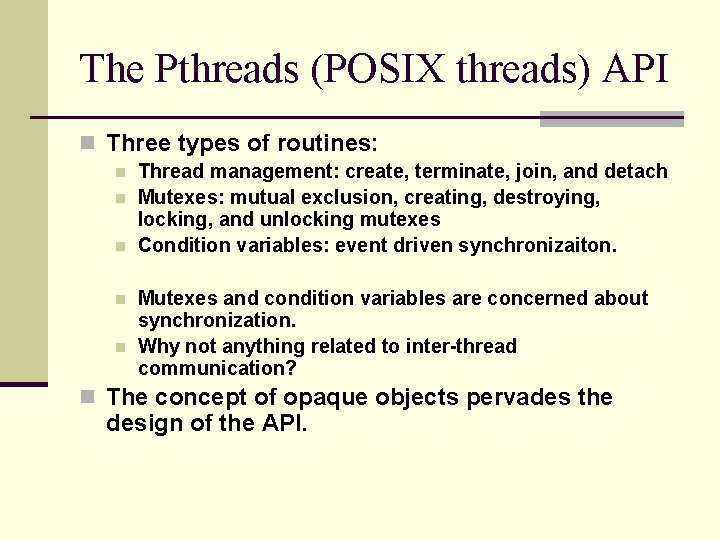The Pthreads (POSIX threads) API n Three types of routines: n n n Thread management: create, terminate, join, and detach Mutexes: mutual exclusion, creating, destroying, locking, and unlocking mutexes Condition variables: event driven synchronizaiton. Mutexes and condition variables are concerned about synchronization. Why not anything related to inter-thread communication? n The concept of opaque objects pervades the design of the API.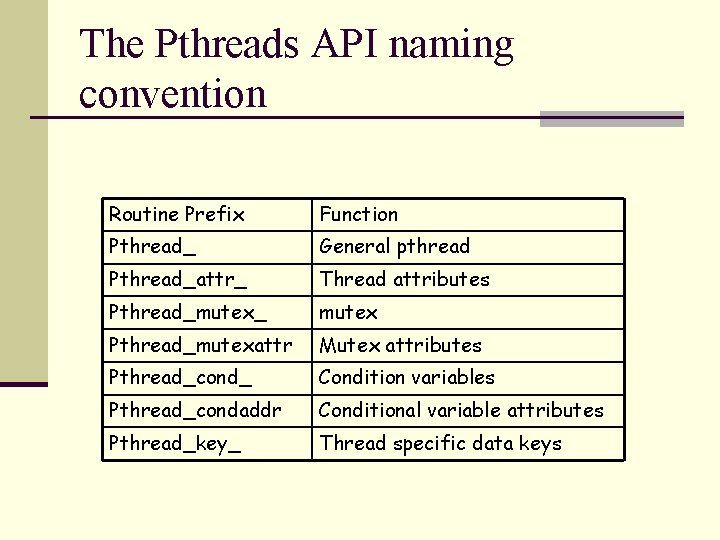The Pthreads API naming convention Routine Prefix Function Pthread_ General pthread Pthread_attr_ Thread attributes Pthread_mutex_ mutex Pthread_mutexattr Mutex attributes Pthread_cond_ Condition variables Pthread_condaddr Conditional variable attributes Pthread_key_ Thread specific data keys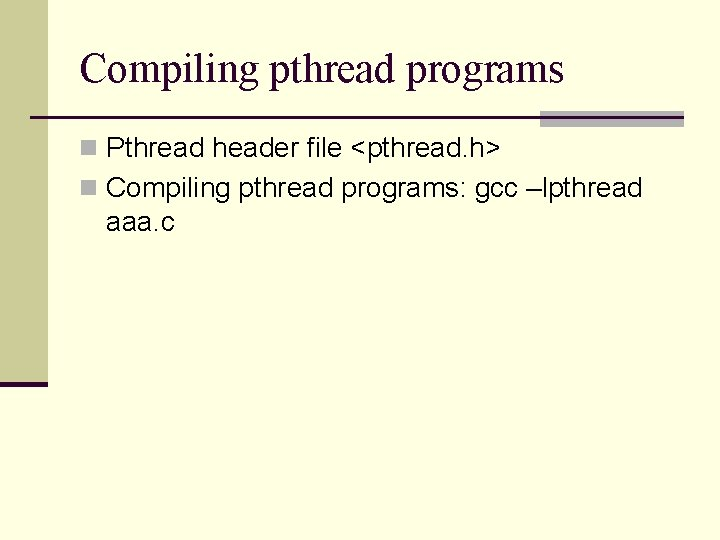Compiling pthread programs n Pthread header file <pthread. h> n Compiling pthread programs: gcc –lpthread aaa. c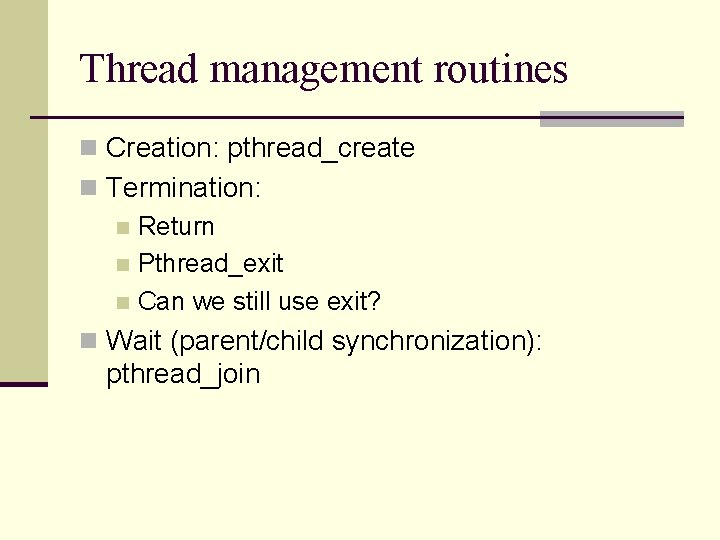Thread management routines n Creation: pthread_create n Termination: n Return n Pthread_exit n Can we still use exit? n Wait (parent/child synchronization): pthread_joinCreation n Thread equivalent of fork() n int pthread_create( pthread_t * thread, pthread_attr_t * attr, void * (*start_routine)(void *), void * arg ); n Returns 0 if OK, and non-zero (> 0) if error. n Parameters for the routines are passed through void * arg. n What if we want to pass a structure?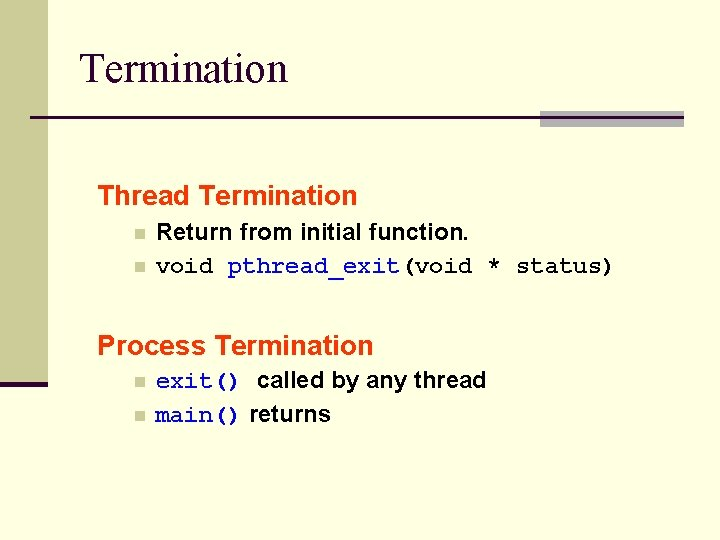Termination Thread Termination n n Return from initial function. void pthread_exit(void * status) Process Termination n n exit() called by any thread main() returns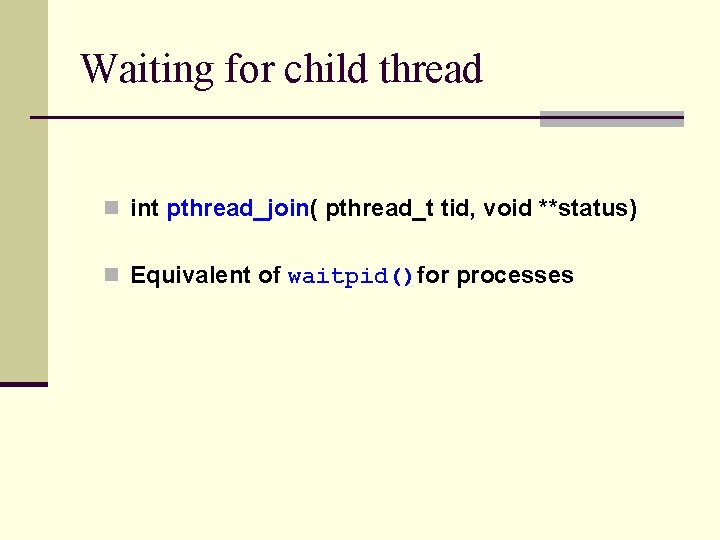Waiting for child thread n int pthread_join( pthread_t tid, void **status) n Equivalent of waitpid()for processes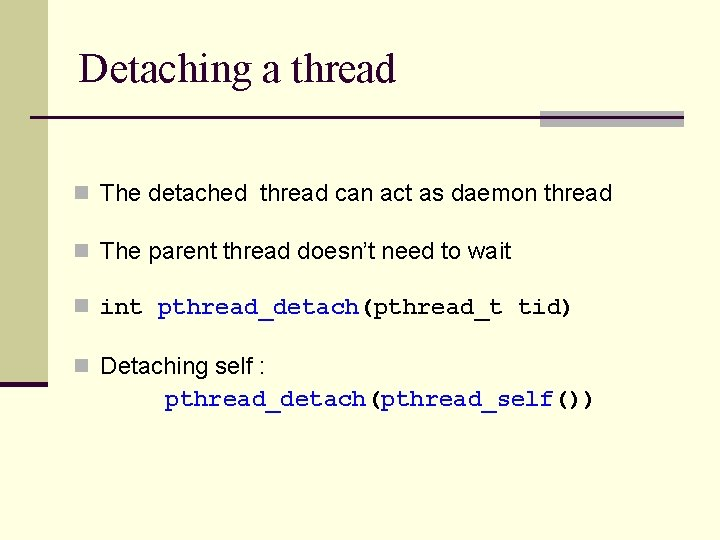Detaching a thread n The detached thread can act as daemon thread n The parent thread doesn’t need to wait n int pthread_detach(pthread_t tid) n Detaching self : pthread_detach(pthread_self())Some multi-thread program examples n A multi-thread program example: example 1. c n Making multiple producers: example 2. c n What is going on in this program? n How to fix it?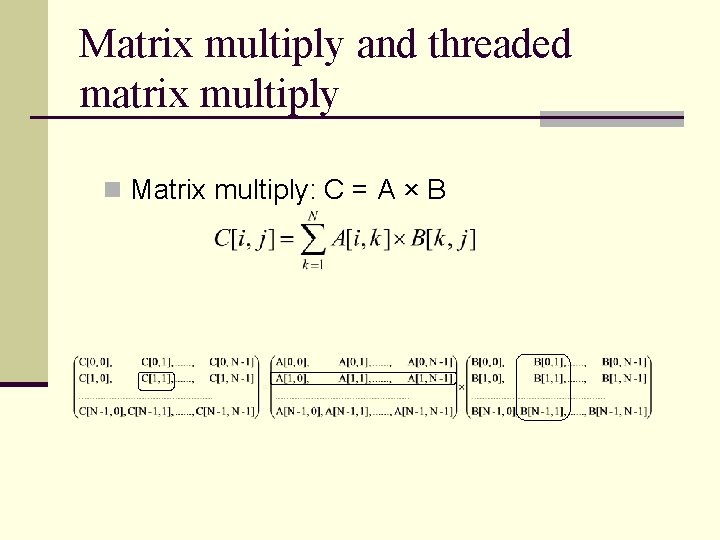Matrix multiply and threaded matrix multiply n Matrix multiply: C = A × BMatrix multiply and threaded matrix multiply n Sequential code: For (i=0; i<N; i++) for (j=0; j<N; j++) for (k=0; k<N; k++) C[I, j] = C[I, j] + A[I, k] * A[k, j] n Threaded code program n The calculation of c[I, j] does not depend on other C term. Mm_pthread. c.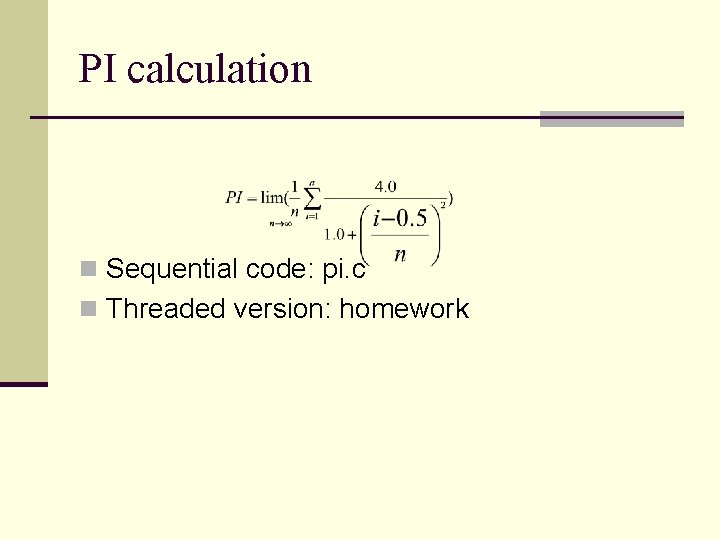PI calculation n Sequential code: pi. c n Threaded version: homework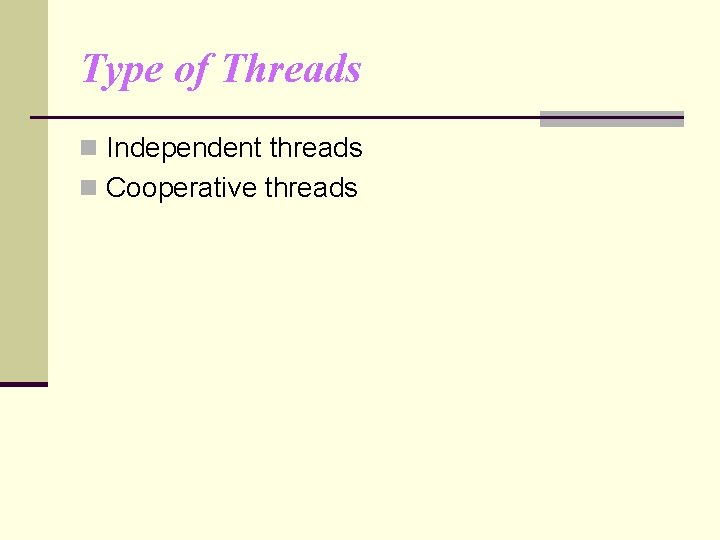Type of Threads n Independent threads n Cooperative threads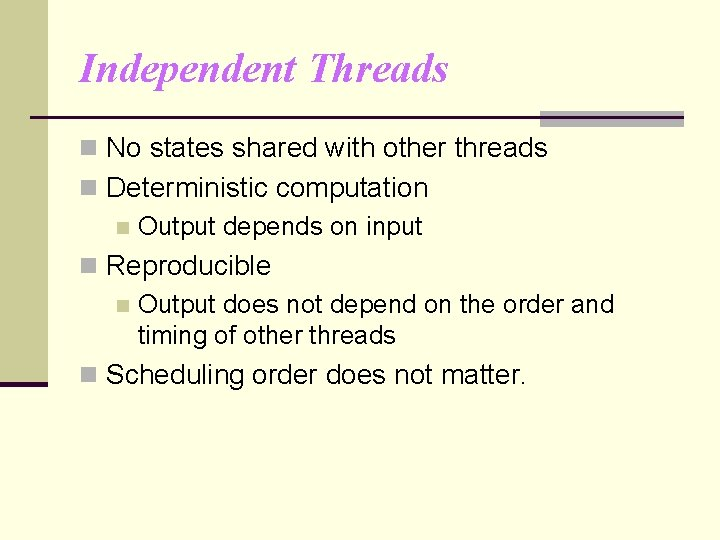Independent Threads n No states shared with other threads n Deterministic computation n Output depends on input n Reproducible n Output does not depend on the order and timing of other threads n Scheduling order does not matter.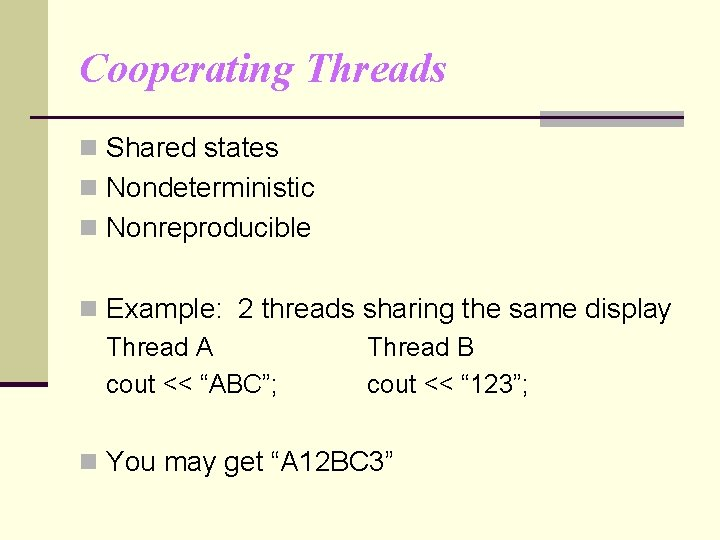Cooperating Threads n Shared states n Nondeterministic n Nonreproducible n Example: 2 threads sharing the same display Thread A Thread B cout << “ABC”; cout << “ 123”; n You may get “A 12 BC 3”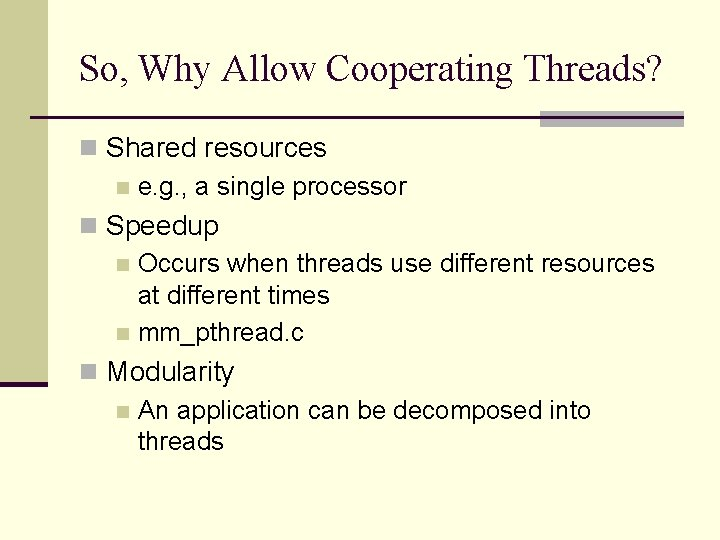So, Why Allow Cooperating Threads? n Shared resources n e. g. , a single processor n Speedup n Occurs when threads use different resources at different times n mm_pthread. c n Modularity n An application can be decomposed into threads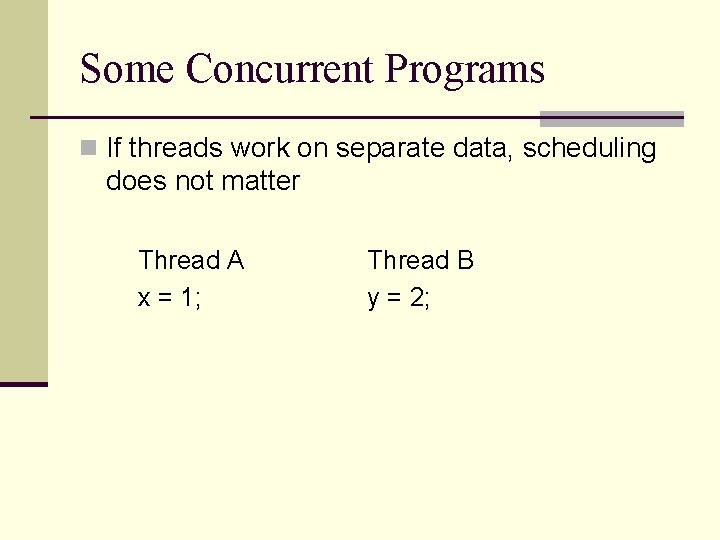Some Concurrent Programs n If threads work on separate data, scheduling does not matter Thread A x = 1; Thread B y = 2;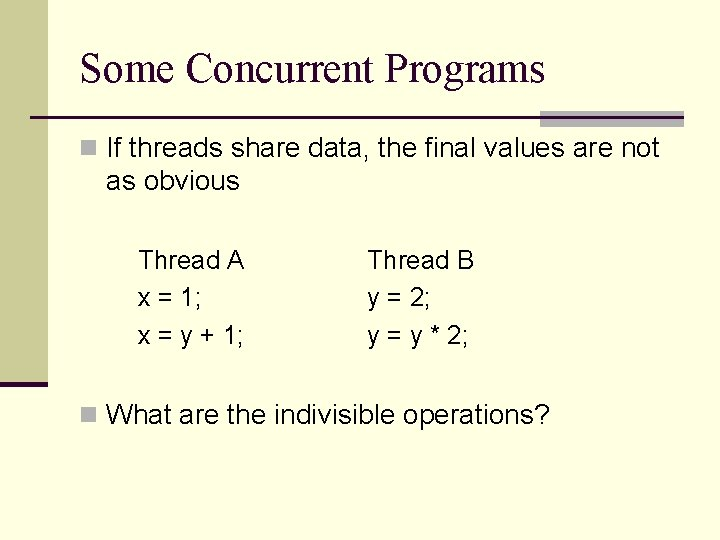Some Concurrent Programs n If threads share data, the final values are not as obvious Thread A x = 1; x = y + 1; Thread B y = 2; y = y * 2; n What are the indivisible operations?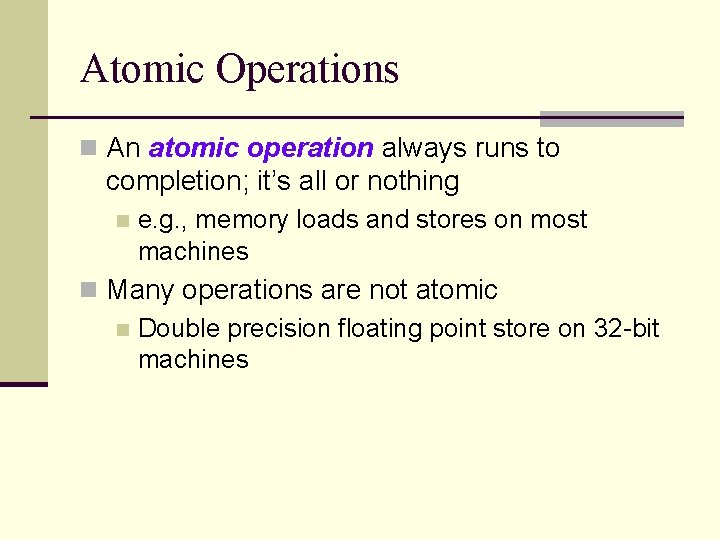Atomic Operations n An atomic operation always runs to completion; it’s all or nothing n e. g. , memory loads and stores on most machines n Many operations are not atomic n Double precision floating point store on 32 -bit machinesSuppose… n Each C statement is atomic n Let’s revisit the example…All Possible Execution Orders Thread A x = 1; x = y + 1; Thread B y = 2; y = y * 2; x=1 x=y+1 y=2 x=1 y=y*2 y=2 x=y+1 y=y*2 x=1 y=y*2 x=y+1 A decision treeAll Possible Execution Orders Thread A x = 1; x = y + 1; Thread B y = 2; y = y * 2; (x = ? , y = ? ) (x = ? , y = 2) y=2 (x = 1, y = ? ) x=1 (x = ? , y = ? ) x=y+1 (x = ? , y = 2) y=2 (x = 1, y = 2) y=2 (x = 3, y = 2) x=y+1 y=y*2 (x = ? , y = 4) (x = 3, y = 4) x=1 (x = ? , y = 4) y=y*2 (x = 1, y = 4) x=1 y=y*2 x=y+1 (x = 5, y = 4)Another Example n Assume each C statement is atomic Thread A j = 0; while (j < 10) { ++j; } cout << “A wins”; Thread B j = 0; while (j > -10) { --j; } cout << “B wins”;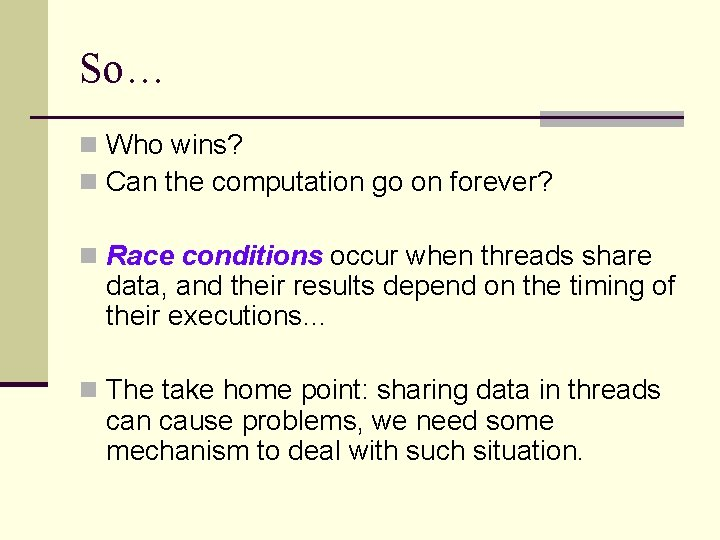So… n Who wins? n Can the computation go on forever? n Race conditions occur when threads share data, and their results depend on the timing of their executions… n The take home point: sharing data in threads can cause problems, we need some mechanism to deal with such situation.